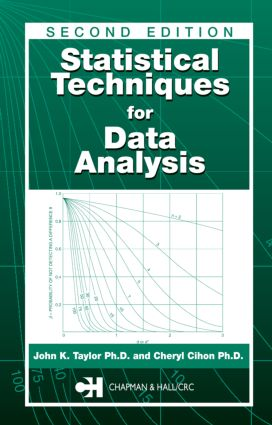Statistical Techniques for Data Analysis

2nd Edition

Chapman and Hall/CRC

296 pages | 42 B/W Illus.

Purchasing Options:\$ = USD
Hardback: 9781584883852
pub: 2004-01-14
SAVE ~\$26.00
Currently out of stock
\$130.00
\$104.00
x
eBook (VitalSource) : 9780429208980
pub: 2004-01-14
from \$28.98

FREE Standard Shipping!

Description

Since the first edition of this book appeared, computers have come to the aid of modern experimenters and data analysts, bringing with them data analysis techniques that were once beyond the calculational reach of even professional statisticians. Today, scientists in every field have access to the techniques and technology they need to analyze statistical data. All they need is practical guidance on how to use them.

Valuable to everyone who produces, uses, or evaluates scientific data, Statistical Techniques for Data Analysis, Second Edition provides straightforward discussion of basic statistical techniques and computer analysis. The purpose, structure, and general principles of the book remain the same as the first edition, but the treatment now includes updates in every chapter, additional topics, and most importantly, an introduction to use of the MINITAB Statistical Software. The presentation of each technique includes motivation and discussion of the statistical analysis, a hand-calculated example, the same example calculated using MINITAB, and discussion of the MINITAB output and conclusions.

Highlights of the Second Edition:

" Detailed discussion and use of MINITAB in examples complete with code and output

" A new chapter addressing proportions, time to event data, and time series data in the metrology setting

" Additional material on hypothesis testing

" Discussion of critical values

" A look at mistakes commonly made in data analysis

WHAT ARE DATA?

Definition of Data

Kinds of Data

Variability

Populations and Samples

Importance of Reliability

Metrology

Computer Assisted Statistical Analyses

Exercises

References

OBTAINING MEANINGFUL DATA

Data Production Must Be Planned

The Experimental Method

Data Quality Indicators

Data Quality Objectives

Systematic Measurement

Quality Assurance

Importance of Peer Review

Exercises

References

GENERAL PRINCIPLES

Introduction

Kinds of Statistics

Decisions

Error and Uncertainty

Kinds of Data

Accuracy, Precision, and Bias

Statistical Control

Distributions

Tests for Normality

Basic Requirements for Statistical Analysis Validity

MINITAB

Exercises

References

STATISTICAL CALCULATIONS

Introduction

The Mean, Variance, and Standard Deviation

Degrees of Freedom

Using Duplicate Measurements to Estimate a Standard Deviation

Using the Range to Estimate the Standard Deviation

Pooled Statistical Estimates

Simple Analysis of Variance

Log Normal Statistics

Minimum Reporting Statistics

Computations

One Last Thing to Remember

Exercises

References

DATA ANALYSIS TECHNIQUES

Introduction

One Sample Topics

Two Sample Topics

Propagation of Error in a Derived or Calculated Value

Exercises

References

MANAGING SETS OF DATA

Introduction

Outliers

Combining Data Sets

Statistics of Interlaboratory Collaborative Testing

Random Numbers

Exercises

References

PRESENTING DATA

Tables

Charts

Graphs

Mathematical Expressions

Exercises

References

PROPORTIONS, SURVIVAL DATA AND TIME SERIES DATA

Introduction

Proportions

Survival Data

Time Series Data

Exercises

References

SELECTED TOPICS

Basic Probability Concepts

Measures of Location

Tests for Nonrandomness

Comparing Several Averages

Type I Errors, Type II Errors and Statistical Power

Critical Values and P Values

Correlation Coefficient

The Best Two Out of Three

Comparing a Frequency Distribution with a Normal Distribution

Confidence for a Fitted Line

Joint Confidence Region for the Constants of a Fitted Line

Shortcut Procedures

Nonparametric Tests

Extreme Value Data

Statistics of Control Charts

Simulation and Macros

Exercises

References

CONCLUSION

Summary

APPENDICES

Statistical Tables

Glossary

Answers to Numerical Exercises

Index